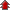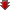# Sequence and Series

• NCERT Chapter### Exercise 1

•  Q1 Write the first five terms of the sequences whose nth term is an=n(n+2) Q2 Write the first five terms of the sequences whose nth term is \begin{align} a_n = \frac {n}{n+1}\end{align}### Exercise 2

•  Q1 Find the sum of odd integers from 1 to 2001. Q2 Find the sum of all natural numbers lying between 100 and 1000, which are multiples of 5. Q3 In an A.P, the first term is 2 and the sum of the first five terms is one-fourth of the next five terms. Show that 20th term is –112. Q4 How many terms of the A.P. \begin{align} -6, -\frac{11}{2}, -5, ... \end{align} are needed to give the sum –25? Q5 In an A.P., if pth term is \begin{align} \frac{1}{q} \; and \;qth\; term \; is\; \frac{1}{p}\end{align} , prove that the sum of first pq terms is \begin{align} \frac{1}{2}(pq+1) \; where \; p ≠ q \end{align} Q6 If the sum of a certain number of terms of the A.P. 25, 22, 19, … is 116. Find the last term Q7 Find the sum to n terms of the A.P., whose kth term is 5k + 1. Q8 If the sum of n terms of an A.P. is (pn + qn2), where p and q are constants, find the common difference Q9 The sums of n terms of two arithmetic progressions are in the ratio 5n + 4: 9n + 6. Find the ratio of their 18th terms. Q10 If the sum of first p terms of an A.P. is equal to the sum of the first q terms, then find the sum of the first (p + q) terms. Q11 ">Sum of the first p, q and r terms of an A.P. are a, b and c, respectively. Prove thatQ12 The ratio of the sums of m and n terms of an A.P. is m2: n2. Show that the ratio of mth and nth term is (2m – 1): (2n – 1). Q13 If the sum of n terms of an A.P. is 3n2 + 5n and its mth term is 164, find the value of m. Q14 Insert five numbers between 8 and 26 such that the resulting sequence is an A.P. Q15 is the A.M. between a and b, then find the value of n.">Ifis the A.M. between a and b, then find the value of n. Q16 Between 1 and 31, m numbers have been inserted in such a way that the resulting sequence is an A.P. and the ratio of 7th and (m – 1)thnumbers is 5:9. Find the value of m. Q17 A man starts repaying a loan as first installment of Rs. 100. If he increases the installment by Rs 5 every month, what amount he will pay in the 30th installment?">A man starts repaying a loan as first installment of Rs. 100. If he increases the installment by Rs 5 every month, what amount he will pay in the 30th installment? Q18 The difference between any two consecutive interior angles of a polygon is 5°. If the smallest angle is 120°, find the number of the sides of the polygon.### Exercise 3

•  Q1 Find the 20th and nthterms of the G.P.">Find the 20th and nthterms of the G.P.Q2 Find the 12th term of a G.P. whose 8th term is 192 and the common ratio is 2. Q3 The 5th, 8th and 11th terms of a G.P. are p, q and s, respectively. Show that q2 = ps. Q4 The 4th term of a G.P. is square of its second term, and the first term is –3. Determine its 7th term. Q5 ">Which term of the following sequences:Q6 are in G.P?">For what values of x, the numbersare in G.P? Q7 Find the sum to 20 terms in the geometric progression 0.15, 0.015, 0.0015 … Q8 "> Find the sum to n terms in the geometric progressionQ9 Find the sum to n terms in the geometric progression 1,-a, a2,-a3, ... (if a ≠ -1)">Find the sum to n terms in the geometric progression 1,-a, a2,-a3, ... (if a ≠ -1) Q10 Find the sum to n terms in the geometric progression x3, x5, x7 ... (if x ≠ ±1)">Find the sum to n terms in the geometric progression x3, x5, x7 ... (if x ≠ ±1) Q11 ">EvaluateQ12 and their product is 1. Find the common ratio and the terms.">The sum of first three terms of a G.P. isand their product is 1. Find the common ratio and the terms. Q13 How many terms of G.P. 3, 32, 33, … are needed to give the sum 120? Q14 The sum of first three terms of a G.P. is 16 and the sum of the next three terms is 128. Determine the first term, the common ratio and the sum to n terms of the G.P. Q15 Given a G.P. with a = 729 and 7th term 64, determine S7. Q16 Find a G.P. for which sum of the first two terms is –4 and the fifth term is 4 times the third term. Q17 If the 4th, 10th and 16th terms of a G.P. are x, y and z, respectively. Prove that x, y, z are in G.P. Q18 Find the sum to n terms of the sequence, 8, 88, 888, 8888…">Find the sum to n terms of the sequence, 8, 88, 888, 8888… Q19 .">Find the sum of the products of the corresponding terms of the sequences 2, 4, 8, 16, 32 and 128, 32, 8, 2,. Q20 Show that the products of the corresponding terms of the sequences a,ar,ar2, ...arn-1 and A, AR, AR2, ,,,ARn-1 form a G.P, and find the common ratio. Q21 Find four numbers forming a geometric progression in which third term is greater than the first term by 9, and the second term is greater than the 4th by 18. Q22 If the pth, qth and rth terms of a G.P. are a, b and c, respectively. Prove that aq-rbr-pcp-q=1 Q23 If the first and the nth term of a G.P. are a ad b, respectively, and if P is the product of n terms, prove that P2 = (ab)n. Q24 Show that the ratio of the sum of first n terms of a G.P. to the sum of terms from">Show that the ratio of the sum of first n terms of a G.P. to the sum of terms fromQ25 If a, b, c and d are in G.P. show that">If a, b, c and d are in G.P. show thatQ26 Insert two numbers between 3 and 81 so that the resulting sequence is G.P. Q27 may be the geometric mean between a and b.">Find the value of n so thatmay be the geometric mean between a and b. Q28 ">The sum of two numbers is 6 times their geometric mean, show that numbers are in the ratioQ29 .">If A and G be A.M. and G.M., respectively between two positive numbers, prove that the numbers are. Q31 What will Rs 500 amounts to in 10 years after its deposit in a bank which pays annual interest rate of 10% compounded annually? Q32 If A.M. and G.M. of roots of a quadratic equation are 8 and 5, respectively, then obtain the quadratic equation.### Exercise 4

•  Q1 Find the sum to n terms of the series 1 × 2 + 2 × 3 + 3 × 4 + 4 × 5 + … Q2 Find the sum to n terms of the series 1 × 2 × 3 + 2 × 3 × 4 + 3 × 4 × 5 + … Q3 Find the sum to n terms of the series 3 × 12 + 5 × 22 + 7 × 32 + … Q4 Find the sum to n terms of the series">Find the sum to n terms of the seriesQ5 Find the sum to n terms of the series 52 + 62 + 72 + ... + 202">Find the sum to n terms of the series 52 + 62 + 72 + ... + 202 Q6 Find the sum to n terms of the series 3 × 8 + 6 × 11 + 9 × 14 +… Q7 Find the sum to n terms of the series 12 + (12 + 22) + (12 + 22 + 32) + … Q8 Find the sum to n terms of the series whose nth term is given by n (n + 1) (n + 4). Q9 Find the sum to n terms of the series whose nth terms is given by n2 + 2n Q10 Find the sum to n terms of the series whose nth terms is given by (2n – 1)2### Exercise 5

•  Q1 Show that the sum of (m + n)th and (m – n)th terms of an A.P. is equal to twice the mth term. Q2 If the sum of three numbers in A.P., is 24 and their product is 440, find the numbers. Q3 Let the sum of n, 2n, 3n terms of an A.P. be S1, S2 and S3, respectively, show that S3 = 3 (S2– S1) Q4 Find the sum of all numbers between 200 and 400 which are divisible by 7. Q5 Find the sum of integers from 1 to 100 that are divisible by 2 or 5. Q6 Find the sum of all two digit numbers which when divided by 4, yields 1 as remainder. Q7 N  such that f(1) = 3and  , find the value of n.">If f is a function satisfying f(x +y) = f(x) f(y) for all x,yN  such that f(1) = 3and  , find the value of n. Q8 The sum of some terms of G.P. is 315 whose first term and the common ratio are 5 and 2, respectively. Find the last term and the number of terms. Q9 The first term of a G.P. is 1. The sum of the third term and fifth term is 90. Find the common ratio of G.P. Q10 The sum of three numbers in G.P. is 56. If we subtract 1, 7, 21 from these numbers in that order, we obtain an arithmetic progression. Find the numbers. Q11 A G.P. consists of an even number of terms. If the sum of all the terms is 5 times the sum of terms occupying odd places, then find its common ratio. Q12 The sum of the first four terms of an A.P. is 56. The sum of the last four terms is 112. If its first term is 11, then find the number of terms. Q13 If [Image_Link]/saralkocadmin/ckeditor_4.4.5/ckeditor//plugins/ckeditor_wiris/in Q14 Let S be the sum, P the product and R the sum of reciprocals of n terms in a G.P. Prove that P2Rn = Sn